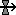#Flow arrow

## Interpretation

The flow arrow is used to specify a term contributing to the rate of change of a compartment. If the flow arrow enters a compartment, it specifies a positive contribution to the rate of change of that compartment. If it leaves the compartment, it specifies a negative contribution to the rate of change.

The information on the flows entering and leaving each compartment is used to calculate the net rate of change of the compartment. The net rate of change is the sum of all the inflow values minus the sum of all the outflow values. The net rate of change is in turn used to calculate the change in the value of the compartment.

If your model needs to keep track of changes in the amount of a substance but you are not interested in where it comes from or goes to, your flow may start or finish on a blank part of the model diagram. In this case a "cloud" will be drawn at the end point, indicating that the amount of substance there plays no role in the model. Each cloud may only have one flow connected to it.

Influences to and from a flow are attached to a "bowtie" (or "valve") symbol which is positioned on the flow. This represents the point that controls the rate of the flow.

In most respects, a flow is treated just like a variable. You can use the full range of the equation language when you enter an equation for the flow, just as you can do for a variable. You can have influence arrows going from it to other parts of the model, again just like a variable. The two differences are that:

• a flow is the only way you can express a rate of change term for a compartment; and
• a flow cannot be a "file parameter".

## Rules

• A flow arrow can only be drawn into and/or out of a compartment.
• Influence arrows can be drawn to and from flows.
• The units for a flow value must be the units of the corresponding compartment(s) that it is linked to, per unit of time. For example, if you have a flow going into a compartment whose units are kg, and the unit of time for the model is a year, then the units for the flow must be kg/year.
• It is quite legitimate to have an influence arrow going from one flow to another. You might wish to do this if one flow is proportional to another, or if the two flows are in different submodels.